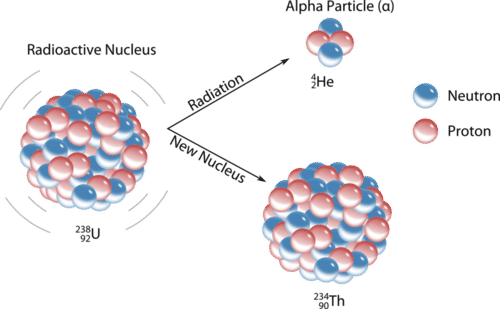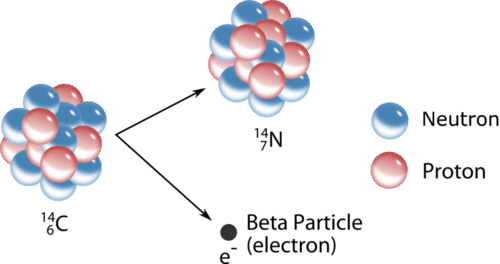11.4: Nuclear Decay

Learning Objectives

• Write and balance nuclear equations
• To know the different kinds of radioactive decay.
• To balance a nuclear reaction.

Unstable nuclei spontaneously emit radiation in the form of particles and energy. This generally changes the number of protons and/or neutrons in the nucleus, resulting in a more stable nuclide. One type of a nuclear reaction is radioactive decay, a reaction in which a nucleus spontaneously disintegrates into a slightly lighter nucleus, accompanied by the emission of particles, energy, or both. An example is shown below, in which the nucleus of a polonium atom radioactively decays into a lead nucleus.

$\ce{^{235}_{92}U \rightarrow \, _2^4He + \, _{90}^{231}Th} \label{Eq2}$

Note that in a balanced nuclear equation, the sum of the atomic numbers (subscripts) and the sum of the mass numbers (superscripts) must be equal on both sides of the equation. How do we know that a product of the reaction is $$\ce{_{90}^{231}Th}$$? We use a modified type of the law of conservation of matter, which says that we must have the same number of protons and neutrons on both sides of the chemical equation. If our uranium nucleus loses 2 protons from the alpha particle, then there are 90 protons remaining, identifying the element as thorium. Moreover, if we lose 4 nuclear particles of the original 235, there are 231 remaining. Thus, we use subtraction to identify the isotope of the thorium atom—in this case, $$\ce{^{231}_{90}Th}$$.

Because the number of protons changes as a result of this nuclear reaction, the identity of the element changes. Transmutation is a change in the identity of a nucleus as a result of a change in the number of protons. There are several different types of naturally occurring radioactive decay, and we will examine each separately.

Alpha Decay

An alpha particle $$\left( \alpha \right)$$ is a helium nucleus with two protons and two neutrons. Alpha particles are emitted during some types of radioactive decay. The net charge of an alpha particle is $$2+$$, and its mass is approximately $$4 \: \text{amu}$$. The symbol for an alpha particle in a nuclear equation is usually $$\ce{^4_2He}$$, though sometimes $$\alpha$$ is used. Alpha decay typically occurs for very heavy nuclei in which the nuclei are unstable due to large numbers of nucleons. For nuclei that undergo alpha decay, their stability is increased by the subtraction of two protons and two neutrons. For example, uranium-238 decays into thorium-234 by the emission of an alpha particle (see figure below).Figure $$\PageIndex{3}$$: The unstable uranium-238 nucleus spontaneously decays into a thorium-234 nucleus by emitting an alpha particle.

Example $$\PageIndex{1}$$: Radon-222

Write the nuclear equation that represents the radioactive decay of radon-222 by alpha particle emission and identify the daughter isotope.

Solution

Radon has an atomic number of 86, so the parent isotope is represented as $$\ce{^{222}_{86}Rn}$$. We represent the alpha particle as $$\ce{^{4}_{2}He}$$ and use subtraction (222 − 4 = 218 and 86 − 2 = 84) to identify the daughter isotope as an isotope of polonium, $$\mathrm{^{218}_{84}Po}$$:

$\ce{_{86}^{222}Rn\rightarrow \, _2^4He + \, _{84}^{218}Po} \nonumber$

Exercise $$\PageIndex{1}$$: Polonium-209

Write the nuclear equation that represents the radioactive decay of polonium-209 by alpha particle emission and identify the daughter isotope.

$\ce{_{84}^{209}Po\rightarrow \, _2^4He + \, _{82}^{205}Pb} \nonumber$

Beta Decay

Nuclei above the band of stability are unstable because their neutron to proton ratio is too high. To decrease that ratio, a neutron in the nucleus is capable of turning into a proton and an electron. The electron is immediately ejected at a high speed from the nucleus. A beta particle $$\left( \beta \right)$$ is a high-speed electron emitted from the nucleus of an atom during some kinds of radioactive decay (see figure below). The symbol for a beta particle in an equation is either $$\beta$$ or $$\ce{^0_{-1}e}$$. Carbon-14 undergoes beta decay, transmutating into a nitrogen-14 nucleus.

$\ce{^{14}_6C} \rightarrow \ce{^{14}_7N} + \ce{^0_{-1}e}$

Note that beta decay increases the atomic number by one, but the mass number remains the same.Figure $$\PageIndex{4}$$: The beta decay of a carbon-14 nuclide involves the conversion of a neutron to a proton and an electron, with the electron being emitted from the nucleus.

Example $$\PageIndex{2}$$: Boron-12

Write the nuclear equation that represents the radioactive decay of boron-12 by beta particle emission and identify the daughter isotope. A gamma ray is emitted simultaneously with the beta particle.

Solution

The parent isotope is $$\ce{^{12}_{5}B}$$ while one of the products is an electron, $$\ce{^{0}_{-1}e}$$. So that the mass and atomic numbers have the same value on both sides, the mass number of the daughter isotope must be 12, and its atomic number must be 6. The element having an atomic number of 6 is carbon. Thus, the complete nuclear equation is as follows:

$\ce{_5^{12}B\rightarrow \, _6^{12}C + \, _{-1}^0e + \gamma} \nonumber$

The daughter isotope is $$\ce{^{12}_6 C}$$.

Exercise $$\PageIndex{2}$$: Iodine-131

Write the nuclear equation that represents the radioactive decay of iodine-131 by beta particle emission and identify the daughter isotope. A gamma ray is emitted simultaneously with the beta particle.

$\ce{_53^{131}I\rightarrow \, _54^{131}Xe + \, _{-1}^0e + \gamma} \nonumber$

Positron Emission

Nuclei below the band of stability are unstable because their neutron to proton ratio is too low. One way to increase that ratio is for a proton in the nucleus to turn into a neutron and another particle called a positron. A positron is a particle with the same mass as an electron, but with a positive charge. Like the beta particle, a positron is immediately ejected from the nucleus upon its formation. The symbol for a positron in an equation is $$\ce{^0_{+1}e}$$. For example, potassium-38 emits a positron, becoming argon-38.

$\ce{^{38}_{19}K} \rightarrow \ce{^{38}_{18}Ar} + \ce{^0_1e}$

Positron emission decreases the atomic number by one, but the mass number remains the same.

Electron Capture

An alternate way for a nuclide to increase its neutron to proton ratio is by a phenomenon called electron capture. In electron capture, an electron from an inner orbital is captured by the nucleus of the atom and combined with a proton to form a neutron. For example, silver-106 undergoes electron capture to become palladium-106.

$\ce{^{106}_{47}Ag} + \ce{^0_{-1}e} \rightarrow \ce{^{106}_{46}Pd}$

Note that the overall result of electron capture is identical to positron emission. The atomic number decreases by one while the mass number remains the same.

Gamma Ray Emission

Gamma rays $$\left( \gamma \right)$$ are very high energy electromagnetic waves emitted from a nucleus. Gamma rays are emitted by a nucleus when nuclear particles undergo transitions between nuclear energy levels. This is analogous to the electromagnetic radiation emitted when excited electrons drop from higher to lower energy levels; the only difference is that nuclear transitions release much more energetic radiation. Gamma ray emission often accompanies the decay of a nuclide by other means.

$\ce{^{230}_{90}Th} \rightarrow \ce{^{226}_{88}Ra} + \ce{^4_2He} + \gamma$

The emission of gamma radiation has no effect on the atomic number or mass number of the products, but it reduces their energy.

The table below summarizes the main types of nuclear radiation, including charge, mass, symbol, and penetrating power. Penetrating power refers to the relative ability of the radiation to pass through common materials. Radiation with high penetrating power is potentially more dangerous because it can pass through skin and do cellular damage.

Table $$\PageIndex{1}$$ Summary of types of nuclear radiation.
Type Symbol Mass number Charge Penetration Power Shielding
Alpha particle $$\ce{^4_2He}$$ or $$\alpha$$ 4 $$2+$$ Low Paper, skin
Beta particle $$\ce{^0_{-1}e}$$ or $$\beta$$ 0 $$1-$$ Moderate Heavy cloth, plastic
Positron $$\ce{^0_1e}$$ or $$\beta^+$$ 0 $$1+$$ Moderate Heavy cloth, plastic
Gamma ray $$\gamma$$ or $$^0_0\gamma$$ 0 0 High Lead, concrete
Neutron $$\ce{^1_0n}$$ 1 0 High Water, lead

Example $$\PageIndex{3}$$

Write a balanced nuclear equation to describe each reaction.

1. the beta decay of $$^{35}_{16}\textrm{S}$$
2. the decay of $$^{201}_{80}\textrm{Hg}$$ by electron capture
3. the decay of $$^{30}_{15}\textrm{P}$$ by positron emission

Given: radioactive nuclide and mode of decay

Strategy:

A Identify the reactants and the products from the information given.

B Use the values of A and Z to identify any missing components needed to balance the equation.

Solution

a.

A We know the identities of the reactant and one of the products (a β particle). We can therefore begin by writing an equation that shows the reactant and one of the products and indicates the unknown product as $$^{A}_{Z}\textrm{X}$$: $^{35}_{16}\textrm{S}\rightarrow\,^{A}_{Z}\textrm{X}+\,^{0}_{-1}\beta \nonumber$

B Because both protons and neutrons must be conserved in a nuclear reaction, the unknown product must have a mass number of A = 35 − 0 = 35 and an atomic number of Z = 16 − (−1) = 17. The element with Z = 17 is chlorine, so the balanced nuclear equation is as follows: $^{35}_{16}\textrm{S}\rightarrow\,^{35}_{17}\textrm{Cl}+\,^{0}_{-1}\beta \nonumber$

b.

A We know the identities of both reactants: $$^{201}_{80}\textrm{Hg}$$ and an inner electron, $$^{0}_{-1}\textrm{e}$$. The reaction is as follows: $^{201}_{80}\textrm{Hg}+\,^{0}_{-1}\textrm e\rightarrow\,^{A}_{Z}\textrm{X} \nonumber$

B Both protons and neutrons are conserved, so the mass number of the product must be A = 201 + 0 = 201, and the atomic number of the product must be Z = 80 + (−1) = 79, which corresponds to the element gold. The balanced nuclear equation is thus $^{201}_{80}\textrm{Hg}+\,^{0}_{-1}\textrm e\rightarrow\,^{201}_{79}\textrm{Au} \nonumber$

c.

A As in part (a), we are given the identities of the reactant and one of the products—in this case, a positron. The unbalanced nuclear equation is therefore $^{30}_{15}\textrm{P}\rightarrow\,^{A}_{Z}\textrm{X}+\,^{0}_{+1}\beta \nonumber$

B The mass number of the second product is A = 30 − 0 = 30, and its atomic number is Z = 15 − 1 = 14, which corresponds to silicon. The balanced nuclear equation for the reaction is as follows: $^{30}_{15}\textrm{P}\rightarrow\,^{30}_{14}\textrm{Si}+\,^{0}_{+1}\beta \nonumber$

Exercise $$\PageIndex{3}$$

Write a balanced nuclear equation to describe each reaction.

1. $$^{11}_{6}\textrm{C}$$ by positron emission
2. the beta decay of molybdenum-99
3. the emission of an α particle followed by gamma emission from $$^{185}_{74}\textrm{W}$$

$$^{11}_{6}\textrm{C}\rightarrow\,^{11}_{5}\textrm{B}+\,^{0}_{+1}\beta$$

$$^{99}_{42}\textrm{Mo}\rightarrow\,^{99m}_{43}\textrm{Tc}+\,^{0}_{-1}\beta$$

$$^{185}_{74}\textrm{W}\rightarrow\,^{181}_{72}\textrm{Hf}+\,^{4}_{2}\alpha +\,^{0}_{0}\gamma$$

Example $$\PageIndex{4}$$

Predict the kind of nuclear change each unstable nuclide undergoes when it decays.

1. $$^{45}_{22}\textrm{Ti}$$
2. $$^{242}_{94}\textrm{Pu}$$
3. $$^{12}_{5}\textrm{B}$$
4. $$^{256}_{100}\textrm{Fm}$$

Given: nuclide

Asked for: type of nuclear decay

Strategy:

Based on the neutron-to-proton ratio and the value of Z, predict the type of nuclear decay reaction that will produce a more stable nuclide.

Solution

1. This nuclide has a neutron-to-proton ratio of only 1.05, which is much less than the requirement for stability for an element with an atomic number in this range. Nuclei that have low neutron-to-proton ratios decay by converting a proton to a neutron. The two possibilities are positron emission, which converts a proton to a neutron and a positron, and electron capture, which converts a proton and a core electron to a neutron. In this case, both are observed, with positron emission occurring about 86% of the time and electron capture about 14% of the time.
2. Nuclei with Z > 83 are too heavy to be stable and usually undergo alpha decay, which decreases both the mass number and the atomic number. Thus $$^{242}_{94}\textrm{Pu}$$ is expected to decay by alpha emission.
3. This nuclide has a neutron-to-proton ratio of 1.4, which is very high for a light element. Nuclei with high neutron-to-proton ratios decay by converting a neutron to a proton and an electron. The electron is emitted as a β particle, and the proton remains in the nucleus, causing an increase in the atomic number with no change in the mass number. We therefore predict that $$^{12}_{5}\textrm{B}$$ will undergo beta decay.
4. This is a massive nuclide, with an atomic number of 100 and a mass number much greater than 200. Nuclides with A ≥ 200 tend to decay by alpha emission, and even heavier nuclei tend to undergo spontaneous fission. We therefore predict that $$^{256}_{100}\textrm{Fm}$$ will decay by either or both of these two processes. In fact, it decays by both spontaneous fission and alpha emission, in a 97:3 ratio.

Exercise $$\PageIndex{4}$$

Predict the kind of nuclear change each unstable nuclide undergoes when it decays.

1. $$^{32}_{14}\textrm{Si}$$
2. $$^{43}_{21}\textrm{Sc}$$
3. $$^{231}_{91}\textrm{Pa}$$

beta decay

positron emission or electron capture